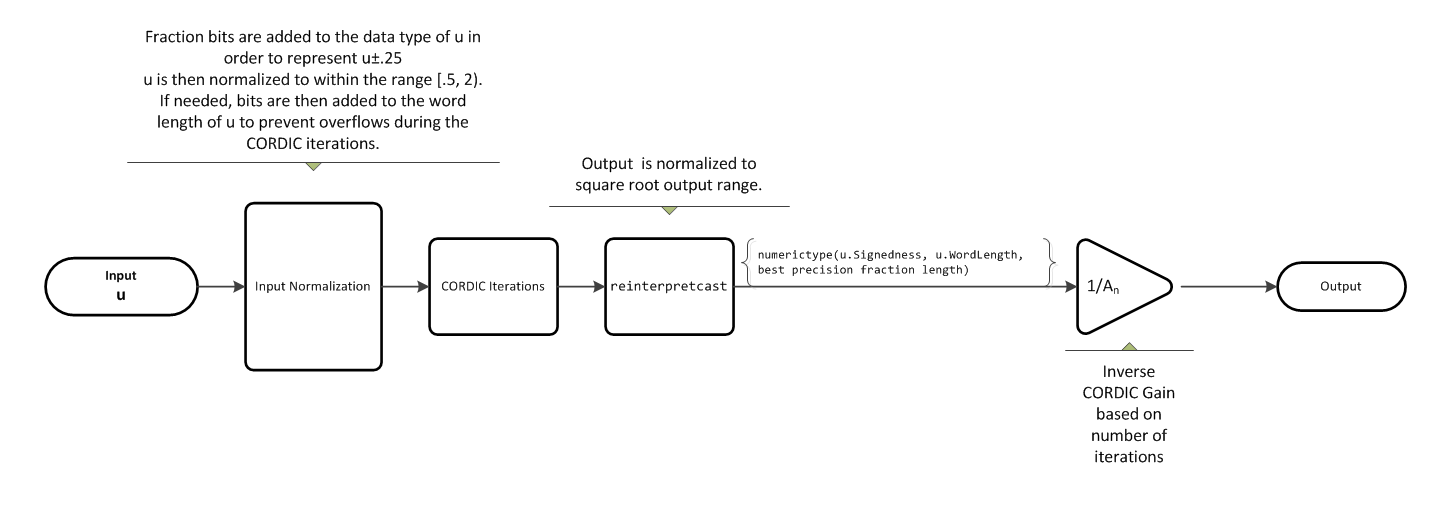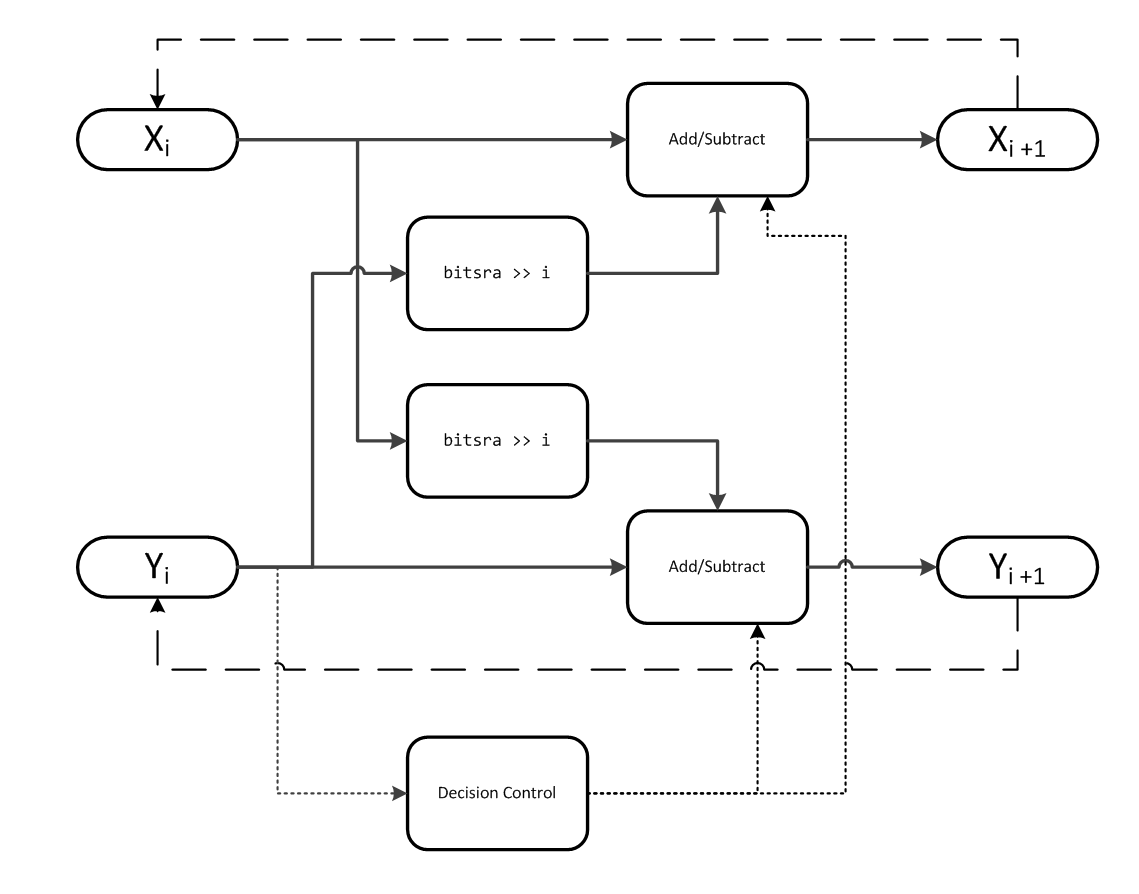このページの翻訳は最新ではありません。ここをクリックして、英語の最新版を参照してください。

# cordicsqrt

## 構文

``y=cordicsqrt(u)``
``y=cordicsqrt(u, niters)``
``y=cordicsqrt(___, 'ScaleOutput', B)``

## 説明

``y=cordicsqrt(u)` は CORDIC アルゴリズムの実装を使用して `u` の平方根を計算します。 `

``y=cordicsqrt(u, niters)` は CORDIC アルゴリズムの `niters` 反復を実行して `u` の平方根を計算します。`

``y=cordicsqrt(___, 'ScaleOutput', B)` では、`B` のブール値に基づいて、出力がスケールされます。`

## 例

すべて折りたたむ

CORDIC の実装を使用して `fi` オブジェクト `x` の平方根を計算します。

```x = fi(1.6,1,12); y = cordicsqrt(x)```
```y = 1.2646 DataTypeMode: Fixed-point: binary point scaling Signedness: Signed WordLength: 12 FractionLength: 10 ```

`niters` を指定しなかったので、関数は最大の反復回数 `x.WordLength - 1` を実行します。

`err = abs(sqrt(double(x))-double(y))`
```err = 1.0821e-04 ```

CORDIC カーネルの 3 回の反復により x の平方根を計算します。

```x = fi(1.6,1,12); y = cordicsqrt(x,3)```
```y = 1.2646 DataTypeMode: Fixed-point: binary point scaling Signedness: Signed WordLength: 12 FractionLength: 10 ```

`err = abs(sqrt(double(x))-double(y))`
```err = 1.0821e-04 ```
```x = fi(1.6,1,12); y = cordicsqrt(x, 'ScaleOutput', 0)```
```y = 1.0479 DataTypeMode: Fixed-point: binary point scaling Signedness: Signed WordLength: 12 FractionLength: 10 ```

`cordicsqrt` アルゴリズムの 10 回の反復の計算結果を、倍精度の関数 `sqrt` の結果と比較します。

```% Create 500 points between [0, 2) stepSize = 2/500; XDbl = 0:stepSize:2; XFxp = fi(XDbl, 1, 12); % signed, 12-bit fixed-point sqrtXRef = sqrt(double(XFxp)); % reference results % Use 12-bit quantized inputs and set the number % of iterations to 10. % Compare the fixed-point CORDIC results to the % double-precision sqrt function results. niters = 10; cdcSqrtX = cordicsqrt(XFxp, niters); errCdcRef = sqrtXRef - double(cdcSqrtX); figure hold on axis([0 2 -.5 1.5]) plot(XFxp, sqrtXRef, 'b') plot(XFxp, cdcSqrtX, 'g') plot(XFxp, errCdcRef, 'r') ylabel('Sqrt(x)') gca.XTick = 0:0.25:2; gca.XTickLabel = {'0','0.25','0.5','0.75','1','1.25','1.5','1.75','2'}; gca.YTick = -.5:.25:1.5; gca.YTickLabel = {'-0.5','-0.25','0','0.25','0.5','0.75','1','1.25','1.5'}; ref_str = 'Reference: sqrt(double(X))'; cdc_str = sprintf('12-bit CORDIC square root; N = %d', niters); err_str = sprintf('Error (max = %f)', max(abs(errCdcRef))); legend(ref_str, cdc_str, err_str, 'Location', 'southeast')```## 入力引数

すべて折りたたむ

データ入力配列。正のスカラー、ベクトル、行列、あるいは固定小数点または組み込みデータ型の多次元配列として指定します。入力配列が 0.5 ～ 2 の値を含む場合に、このアルゴリズムは最も正確になります。事前正規化と事後正規化プロセスは、この範囲外の入力値に対して実行されます。このプロセスの詳細は、事前正規化と事後正規化を参照してください。

データ型: `fi`|`single` | `double` | `int8` | `int16` | `int32` | `int64` | `uint8` | `uint16` | `uint32` | `uint64`

CORDIC アルゴリズムを実行する反復回数。正の整数値スカラーとして指定します。`niters` を指定しない場合、アルゴリズムは既定値を使用します。固定小数点入力では、`niters` の既定値は `u.WordLength - 1` です。浮動小数点入力では、`niters` の既定値は倍精度では 52、単精度では 23 です。

データ型: `fi`|`single` | `double` | `int8` | `int16` | `int32` | `int64` | `uint8` | `uint16` | `uint32` | `uint64`

### 名前と値のペアの引数

オプションのコンマ区切りされた `Name,Value` の引数ペアを指定します。ここで、`Name` は引数名、`Value` は対応する値です。`Name` は引用符で囲まなければなりません。`Name1,Value1,...,NameN,ValueN` のように、複数の名前と値のペアの引数を任意の順序で指定できます。

データ型: `logical`

すべて折りたたむ

## 詳細

すべて折りたたむ

### CORDIC

CORDIC は、COordinate Rotation DIgital Computer の略語です。ギブンス回転に基づく CORDIC アルゴリズムは、Shift-Add 反復演算のみを必要とするため、ハードウェア効率が最も優れたアルゴリズムの 1 つです (参考文献を参照)。CORDIC アルゴリズムは、明示的な乗数を必要としません。CORDIC を使用すると、正弦関数、余弦関数、逆正弦関数、逆余弦関数、逆正接関数、ベクトル振幅関数などのさまざまな関数を計算できます。また、このアルゴリズムは除算、平方根、双曲線、対数などの関数にも使用できます。

CORDIC の反復数を増やすと、結果の精度が高まりますが、それにより計算量が増加しレイテンシが増えます。

## アルゴリズム

すべて折りたたむ

### 信号の流れ図CORDIC 双曲線カーネルX は `u'+.25` に初期化され、Y は `u'-.25` に初期化されます。ここで、`u'` は正規化された関数入力です。

CORDIC 双曲線カーネルを繰り返し反復することで、X は ${A}_{N}\sqrt{u\text{'}}$ に近づきます。ここで、AN は CORDIC ゲインを表します。Y は `0` に近づきます。

### 事前正規化と事後正規化

[0.5, 2) の範囲外の入力値には、事前および事後正規化プロセスが発生します。このプロセスでは、入力配列に対してビットシフトを実行し、その後 CORDIC カーネルに渡します。その後、事後正規化段階中に結果が正しい出力範囲に戻ります。このプロセスの詳細については、CORDIC を使用した平方根の計算の「アルゴリズム入力範囲制限の回避」を参照してください。

### fimath の伝播ルール

CORDIC 関数は、入力に追加されたすべてのローカル `fimath` を破棄します。

CORDIC 関数は、計算の実行時に独自の内部 `fimath` を使用します。

• `OverflowAction``Wrap`

• `RoundingMethod``Floor`

 Volder, JE. “The CORDIC Trigonometric Computing Technique.” IRE Transactions on Electronic Computers. Vol. EC-8, September 1959, pp. 330–334.

 Andraka, R. “A survey of CORDIC algorithm for FPGA based computers.” Proceedings of the 1998 ACM/SIGDA sixth international symposium on Field programmable gate arrays. Feb. 22–24, 1998, pp. 191–200.

 Walther, J.S. “A Unified Algorithm for Elementary Functions.” Hewlett-Packard Company, Palo Alto. Spring Joint Computer Conference, 1971, pp. 379–386. (from the collection of the Computer History Museum). www.computer.org/csdl/proceedings/afips/1971/5077/00/50770379.pdf

 Schelin, Charles W. “Calculator Function Approximation.” The American Mathematical Monthly. Vol. 90, No. 5, May 1983, pp. 317–325.

## サポート